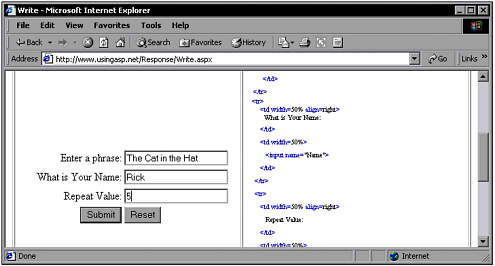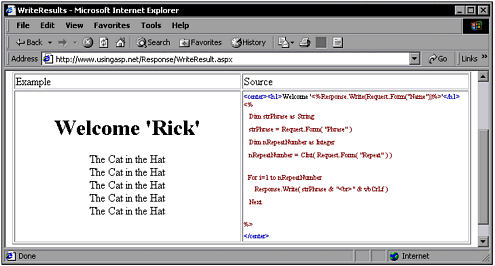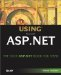Write

Write

The most obvious requirement for the Response object is for it to send a page back to the client browser containing text, graphics, and other interesting content that visitors expect. As mentioned in Chapter 3, the following syntax sends the contents of a string variable out as part of the HTML document:

<%=strSomeTextData%>

This actually is a shortened nomenclature for the Response.Write() method. The following has exactly the same result, but uses the unabridged syntax:

Response.Write( strSomeTextData )

The abbreviated syntax can become more cumbersome for complex expressions than simply outputting a single string variable. When your code needs to write complex expressions to the HTML document, using the Response.Write() method makes code easier to read and trace. The following example shows a more complex output expression:

Response.Write( "Here is some text:" + strSomeTextData + "<br" + vbCrLf )

This is just like real programming. You can construct strings in code and write them to the page. The following two code fragments produce exactly the same output:

<%    Dim nNumber as Integer    For nNumber=1 to 6  %>      The number is: <%=nNumber.ToString()%><br>  <%    Next  %>  <%    Dim nNumber as Integer    For nNumber=1 to 6      Dim strOutput as String      strOutput = "The number is: " + nNumber.ToString() + "<br>"      Response.Write( strOutput )    Next  %>

Note

This code is part of the page that you can find at www.UsingASP.net. Select Chapter Examples, Chapter 4, then Simple Output Loop. You can see the page in Figure 4.1.

Figure 4.1. This is the result of the code.As you create more complex code, the ratio of code to HTML increases ”and using the Response.Write() method helps make code more readable.

The Response.Write() method automatically converts the text to an appropriate character set when it is sent. If you don't want a character set translation, you should use the Response.BinaryWrite() method instead.

A more complex example follows . It asks for the user 's name , a phrase, and a repeat number. These requests are in a form, and their contents will be readily usable in the page to which the form will be submitted.

<form method="POST" action="WriteResults.aspx">    Enter a phrase:<br>    <input name="Phrase"><br>    What is Your Name:<br>    <input name="Name"><br>    Repeat Value:<br>    <input name="Repeat"><br>    <input type="submit" value="Submit" name="B1">    <input type="reset" value="Reset" name="B2">  </form>

Note

This code is part of the page that you can find at www.UsingASP.net. (As with examples in Chapter 3, the formatting tags have been removed here in the text for clarity.) Select Chapter Examples, Chapter 4, then Write. You can see the page in Figure 4.2.

Figure 4.2. This demo asks for a repeat value.When the form is submitted, the repeat number is evaluated using the Convert.ToInt32() method. This method converts a string value to an integer. The following code fragment shows how Convert.ToInt32() can be used:

Dim strValue as String  Dim nValue as Integer  strValue = "34"  nValue = Convert.ToInt32( strValue )  ' nValue now contains an integer value of 34

The following example code loops through and outputs the phrase as many times as the user specified:

<center>    <h1>Welcome '<%Response.Write(Request.Form("Name"))%>'</h1>    <%      Dim strPhrase As String      strPhrase = Request.Form( "Phrase" )      Dim nRepeatNumber As Integer      nRepeatNumber = Convert.ToInt32( Request.Form( "Repeat" )      Dim i As Integer      For i=1 to nRepeatNumber        Response.Write( strPhrase & "<br>" & vbCrLf )      Next    %>  </center>

Note

This code is part of the page that is invoked by filling out the form shown in Figure 4.2 and clicking the Submit button. You can see the rendered page in Figure 4.3.

Figure 4.3. Here the string is written the specified number of times.Special Edition Using ASP.Net
ISBN: 0789725606
EAN: 2147483647
Year: 2002
Pages: 233